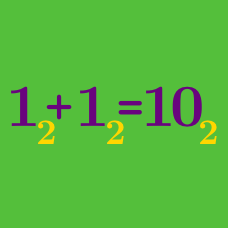Number Theory

# Number Bases Warmup

$11 + 1 = 100$ In which base is this equation true?

$66 + 66 = 143$ In which base is this equation true?

$12 \times 3 = 40$ In which base is this equation true?

$12 \times 9 = A2$ In which base is this equation true?

Note: In this equation, $A$ is a digit representing the number written as 10 in base 10.

$88 + 8 = 90$ In which base is this equation true?

×